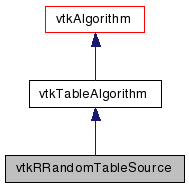# vtkRRandomTableSource Class Reference

`#include <vtkRRandomTableSource.h>`

Inheritance diagram for vtkRRandomTableSource:[legend]
Collaboration diagram for vtkRRandomTableSource:[legend]

## Detailed Description

Generates vtkTables with columns of random numbers using Gnu R.

Note - An installation of Gnu R is required to build and use this class.

vtkRRandomTableSource uses the Gnu R math C language API for statistical distributions to generate vtkTables with columns of random numbers selected from a chosen distribution. The available distributions are as follows:

Normal, Cauchy, F, Student T, Poisson, Chi-Square, Exponential, Binomial, Beta, Geometric, Uniform, Gamma, Log-Normal, Logistic, Hyper-Geometric, Weibull, Negative Binomial, Wilcox

The default output for the class is a table of one column with ten rows of random numbers chosen from a Normal distribution of mean 0.0 and standard deviation of 1.0.

Use SetNumberOfRows() to set the number of rows (random numbers) in the output table.

See comments for SetStatisticalDistributionForColumn() to set the distribution output for a particular column or all columns in the output table.

vtkRInterface
Thanks:
Developed by Thomas J. Otahal (tjotaha@sandia.gov) at Sandia National Laboratories.
Examples:
vtkRRandomTableSource (Examples)
Tests:
vtkRRandomTableSource (Tests)

Definition at line 64 of file vtkRRandomTableSource.h.

enum  StatDistType {
WILCOXONRANKSUM = 0, WILCOXONSIGNEDRANK = 1, LOGISTIC = 2, WEIBULL = 3,
POISSON = 4, NEGBINOMIAL = 5, HYPERGEOM = 6, GEOM = 7,
EXP = 8, CAUCHY = 9, T = 10, F = 11,
LOGNORMAL = 12, GAMMA = 13, UNIF = 14, BETA = 15,
BINOMIAL = 16, NORMAL = 17, CHISQUARE = 18
}
void SetStatisticalDistributionForColumn (vtkRRandomTableSource::StatDistType t, double param1, double param2, double param3, const char *ColumnName, int column_index)

## Public Types

typedef vtkTableAlgorithm Superclass

## Public Member Functions

virtual const char * GetClassName ()
virtual int IsA (const char *type)
void PrintSelf (ostream &os, vtkIndent indent)
int GetNumberOfColumns ()
void ClearTableOutput ()
void SetRandGenSeed (const int seed)
void SetNumberOfRows (int nrows)
int GetNumberOfRows ()
void SetStatisticalDistributionForColumn (int StatDistType, double param1, double param2, double param3, const char *ColumnName, int column_index)

## Static Public Member Functions

static vtkRRandomTableSourceNew ()
static int IsTypeOf (const char *type)
static vtkRRandomTableSourceSafeDownCast (vtkObject *o)

## Protected Member Functions

vtkRRandomTableSource ()
~vtkRRandomTableSource ()
int RequestData (vtkInformation *, vtkInformationVector **, vtkInformationVector *)

## Member Typedef Documentation

Reimplemented from vtkTableAlgorithm.

Definition at line 70 of file vtkRRandomTableSource.h.

## Member Enumeration Documentation

Available statistical distribution output types. Depending on the distribution type, up to three parameters (param1, param2, param3) must be specified when using SetStatisticalDistributionForColumn().

Enumerator:
 WILCOXONRANKSUM WILCOXONSIGNEDRANK LOGISTIC WEIBULL POISSON NEGBINOMIAL HYPERGEOM GEOM EXP CAUCHY T F LOGNORMAL GAMMA UNIF BETA BINOMIAL NORMAL CHISQUARE

Definition at line 95 of file vtkRRandomTableSource.h.

## Constructor & Destructor Documentation

 vtkRRandomTableSource::vtkRRandomTableSource ( ) ` [protected]`

 vtkRRandomTableSource::~vtkRRandomTableSource ( ) ` [protected]`

## Member Function Documentation

 static vtkRRandomTableSource* vtkRRandomTableSource::New ( ) ` [static]`

Create an object with Debug turned off, modified time initialized to zero, and reference counting on.

Reimplemented from vtkTableAlgorithm.

 virtual const char* vtkRRandomTableSource::GetClassName ( ) ` [virtual]`

Reimplemented from vtkTableAlgorithm.

 static int vtkRRandomTableSource::IsTypeOf ( const char * name ) ` [static]`

Return 1 if this class type is the same type of (or a subclass of) the named class. Returns 0 otherwise. This method works in combination with vtkTypeMacro found in vtkSetGet.h.

Reimplemented from vtkTableAlgorithm.

 virtual int vtkRRandomTableSource::IsA ( const char * name ) ` [virtual]`

Return 1 if this class is the same type of (or a subclass of) the named class. Returns 0 otherwise. This method works in combination with vtkTypeMacro found in vtkSetGet.h.

Reimplemented from vtkTableAlgorithm.

 static vtkRRandomTableSource* vtkRRandomTableSource::SafeDownCast ( vtkObject * o ) ` [static]`

Reimplemented from vtkTableAlgorithm.

 void vtkRRandomTableSource::PrintSelf ( ostream & os, vtkIndent indent ) ` [virtual]`

Methods invoked by print to print information about the object including superclasses. Typically not called by the user (use Print() instead) but used in the hierarchical print process to combine the output of several classes.

Reimplemented from vtkTableAlgorithm.

 void vtkRRandomTableSource::SetNumberOfRows ( int nrows )

Set and Get the number of rows in the output table (random numbers).

 int vtkRRandomTableSource::GetNumberOfRows ( )

Set and Get the number of rows in the output table (random numbers).

 int vtkRRandomTableSource::GetNumberOfColumns ( )

Returns number of columns in the output table.

 void vtkRRandomTableSource::ClearTableOutput ( )

Clears table output to zero output columns. Number of table rows is unchanged.

 void vtkRRandomTableSource::SetRandGenSeed ( const int seed )

Set the random seed used by Gnu R to generate output. The default is to use the random seed provided by Gnu R based on the current time.

 void vtkRRandomTableSource::SetStatisticalDistributionForColumn ( vtkRRandomTableSource::StatDistType t, double param1, double param2, double param3, const char * ColumnName, int column_index )

Set the statistical distribution to generate random numbers for a particular column or all columns in the output table. Use the above documented distribution types, for example use vtkRRandomTableSource::Normal for a Normal distribution. Set unused parameter values to 0.0. For example, a Normal distribution uses only param1 and param2 as the mean and the standard deviation respectively. Set param3 to 0.0. If column_index equals the current number of columns in the output table, a new column will be added to the output table and initialized with the input distribution parameters.

 void vtkRRandomTableSource::SetStatisticalDistributionForColumn ( int StatDistType, double param1, double param2, double param3, const char * ColumnName, int column_index )

Python wrapped version of above method. Use integer equivalent of StatDistType.

 int vtkRRandomTableSource::RequestData ( vtkInformation * request, vtkInformationVector ** inputVector, vtkInformationVector * outputVector ) ` [protected, virtual]`

This is called by the superclass. This is the method you should override.

Reimplemented from vtkTableAlgorithm.

The documentation for this class was generated from the following file:

Generated on Wed Aug 24 12:04:27 2011 for VTK by1.5.6